# ISODD Function

Returns TRUE if the number is odd or FALSE if the number is even

## What is the ISODD Function?

The ISODD Function is categorized under Excel Information functions. ISODD returns TRUE if the number is odd or FALSE if the number is even. It is a reference function that helps to indicate if a given number is odd or not.

While doing financial analysis, we need to deal with numbers. The ISODD function is useful in data analysis where we need to identify even or odd numbers.

### Formula

=ISODD(number)

The ISODD function uses the following argument:

1. Number (required argument) – This is the value we wish to test. If it is not an integer, the value is truncated.

### How to use the ISODD Function in Excel?

ISODD is a built-in function in Excel and can be used as a worksheet function in Excel. As a worksheet function, it can be entered as part of a formula in a cell of a worksheet.

To understand the uses of this function, let’s consider a few examples:

#### Example 1

Let’s see the results from this function when we provide the following data: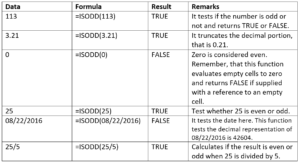The formula used and results in Excel are shown below: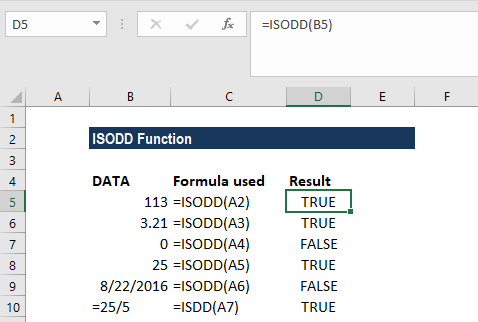#### Example 2

Suppose we are given the following data: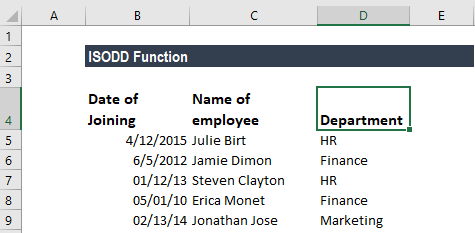We wish to highlight all odd rows in the table above. We can do this using conditional formatting. The formula to be used here would be ISODD. For example, as we wish to highlight the odd rows, first, we will select the entire range of data and then create conditional formatting rules that will use the formula:

=ISODD(ROW(A1:C6))

How to create conditional formatting:

Under the Home tab – Styles, click on Conditional Formatting. Then click on New Rule and then select, “Use a Formula to determine which cells to format”: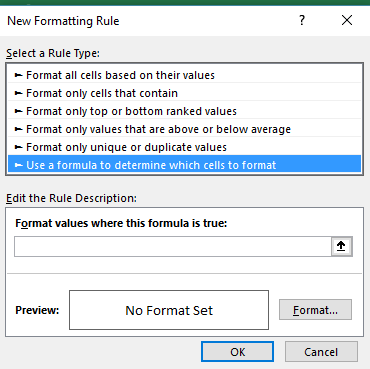The input formula is shown below:The results are shown below: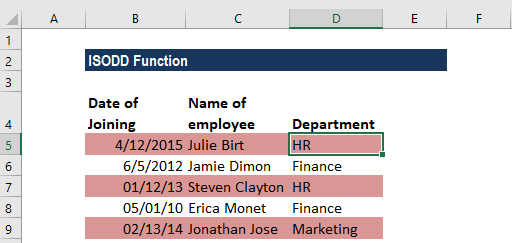How the formula worked:

When we use a formula to apply conditional formatting, the formula will evaluate every cell in the selected range. As there are no addresses in the formula, for every cell in the data the ROW and ISODD functions are run. ROW will return the row number of the cell, and ISODD will return TRUE if the row number is odd and FALSE if the row number is even. The rule will trigger on TRUE, so the even rows will be shaded in this case, as specified by us.

If we wish to color even rows, we can use ISEVEN function in that scenario.

### Things to remember about the ISODD Function

1. #VALUE! error – Occurs when the given argument is non-numeric.
2. We can insert a reference to a cell as seen in the examples above, or a number directly in double quotes to get the desired result.
3. The ISODD function was introduced in Excel 2007 and, hence, is unavailable in earlier versions. It will return an error if used in earlier versions. For older versions, we can use the MOD function.
4. ISODD is the opposite of the ISEVEN function.

Thanks for reading CFI’s guide to important Excel functions! By taking the time to learn and master these functions, you’ll significantly speed up your financial analysis. To learn more, check out these additional CFI resources:

• Excel Functions for Finance